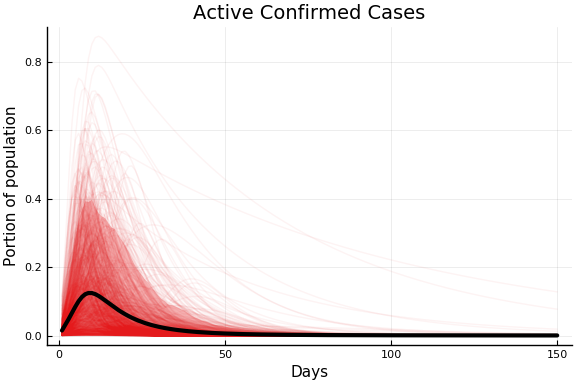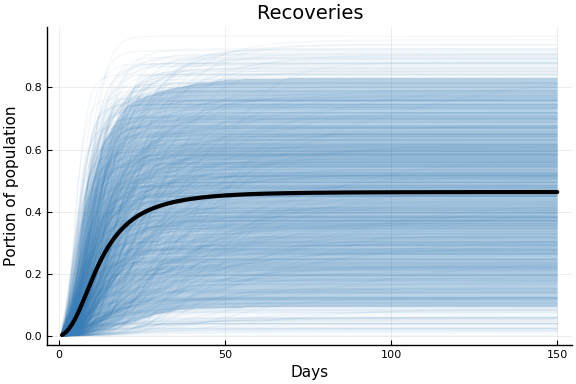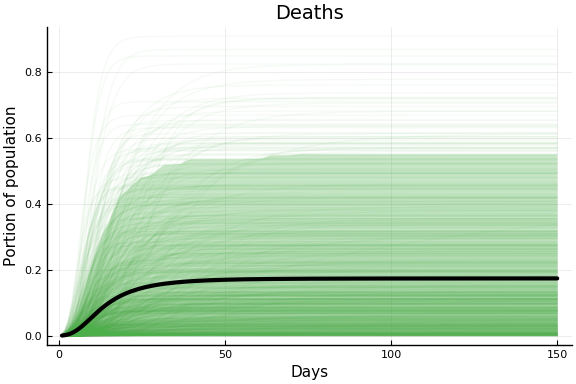using CovidSEIR
using Plots
Plots.pyplot()
using DataFrames, JLD2
jmddir = normpath(joinpath(dirname(Base.find_package("CovidSEIR")),"..","docs","jmd"))

"/home/paul/.julia/dev/CovidSEIR/docs/jmd"


# Data¶

We will use data from Johns Hopkins University Center for Systems Science and Engineering. It is gathered from a variety of sources and updated daily. JHU CSSE uses the data for this interactive website.. For another course, I wrote some notes using this data in python here.

This data has daily cumulative counts of confirmed cases, recoveries, and deaths in each country (and provinces within some countries).

covdf = covidjhudata()
describe(covdf)

11×8 DataFrames.DataFrame. Omitted printing of 2 columns
│ Row │ variable  │ mean      │ min         │ median    │ max        │ nuni
que │
│     │ Symbol    │ Union…    │ Any         │ Union…    │ Any        │ Unio
n…  │
├─────┼───────────┼───────────┼─────────────┼───────────┼────────────┼─────
────┤
│ 1   │ Date      │           │ 2020-01-22  │           │ 2020-04-15 │ 85
│
│ 2   │ confirmed │ 1481.88   │ -1          │ 2.0       │ 636350     │
│
│ 3   │ Province  │           │ Alberta     │           │ Zhejiang   │ 82
│
│ 4   │ Country   │           │ Afghanistan │           │ Zimbabwe   │ 185
│
│ 5   │ Lat       │ 21.1709   │ -51.7963    │ 22.3      │ 71.7069    │
│
│ 6   │ Long      │ 21.7781   │ -135.0      │ 20.903    │ 178.065    │
│
│ 7   │ deaths    │ 78.9457   │ -1          │ 0.0       │ 28326      │
│
│ 8   │ recovered │ 381.939   │ 0           │ 0.0       │ 72600      │
│
│ 9   │ iso2c     │           │ AD          │           │ ZW         │ 178
│
│ 10  │ cpop      │ 2.25233e8 │ 33785.0     │ 2.49924e7 │ 1.39273e9  │
│
│ 11  │ ppop      │ 2.74801e7 │ 41078       │ 1.557e7   │ 111690000  │
│


# Model¶

We will estimate a susceptible, exposed, infectious, recovered (SEIR) epidemiological model of Covid transmission. In particular, we will use a version based on this webapp by Allison Hill.

The model contains the following variables, all of which are functions of time

• $S$: Susceptible individuals
• $E$: Exposed individuals - infected but not yet infectious or symptomatic
• $I_i$: Undetected infected individuals in severity class $i$. Severity increaes with $i$ and we assume individuals must pass through all previous classes
• $I_1$: Mild infection
• $I_2$: Severe infection
• $C_i$ confirmed infected individuals in severity class $i$
• $R = R_u + R_c$: individuals who have recovered from disease and are now immune
• $R_u$ recovered individuals whose infection were never detected
• $R_c$ recovered individuals who were confirmed cases
• $X$: Dead individuals

Compared to Hill’s model, we have reduced the number of severity classes and from 3 to 2, and we have added undetected infections and recoveries. In the data, we observe active confirmed cases $\approx \sum_i C_i$, deaths $\approx X$, and confirmed recoveries $\approx R_c$.

These variables evolve according to the following system of differential equations.

Where the parameters are :

• $\beta_1$, $\beta_2$ baseline rate at which confirmed infected individuals in class $i$ contact susceptibles and infect them
• $\beta_1+\beta_3$ baseline rate at which undetected infected individuals infect others
• $R(t)$ reduction in infection rate due to isolation, quarantine, and/or lockdown
• $a$ rate of progression from the exposed to infected class
• $\gamma_i$ rate at which infected individuals in class $i$ recover from disease and become immune
• $p_1$ rate at which infected individuals in class $i$ progress to class $i+1$
• $p_2$ death rate for individuals in the most severe stage of disease
• $\tau$ rate at which infections of class $1$ are detected

Note that we are assuming that all severe infections are detected (and hence $I_2 = 0$). We are also assuming that confirmed and unconfirmed cases have the same recovery and progression rates.

## Empirical Model¶

Our data has country population, $N$, daily cumulative confirmed cases, $c_t$, deaths, $d_t$, and recoveries, $r_t$. We will assume that at a known time 0, there is an unknown portion of exposed individuals, $p_0$, so

and all other model variables are 0 at time 0. We assume that the observed data is distributed as

We parameterize the reduction in infection rates from public policy as

This implies that infection rates drop by roughly 100$\rho_1$ percent in the week centered on $t=\rho_2$.

## Model Limitations¶

An important limitation is that the model assumes all other parameters are constant over time. Although we allow changes in the infection rate, efforts have also been made to increase $\tau$. Innovations in treatment and crowding of the medical system likely lead to variation in $\gamma$ and $p$.

# Single Country Estimates¶

## Priors¶

We use the follow prior distributions. The means of these are loosely based on Hill’s defaults.

using Distributions
defaultcountrypriors() = Dict(
"a" => truncated(Normal(1/5, 3), 1/14, 1.0),
"p" => truncated(Normal(0.05, 0.3), 0, 1),
"p" => truncated(Normal(0.05, 0.3), 0, 1),
"γ" => truncated(Normal(0.133, 0.5), 0, 3),
"γ" => truncated(Normal(0.05, 0.3), 0, 1),
"β" => truncated(Normal(0.5, 1), 0, 10),
"β" => truncated(Normal(0.5, 1), 0, 10),
"τ" => truncated(Normal(0.2, 2), 0, 10),
"pE0" => truncated(Normal(0.001, 0.1), 0, 1),
"sigD" => InverseGamma(2,3),
"sigC" => InverseGamma(2,3),
"sigRc" => InverseGamma(2,3),
"ρ" => truncated(Normal(0.5, 2), 0, 1),
"ρ" => truncated(Normal(30, 30), 0, 100)


Summary statistics of draws from this prior distribution are below.

priors = CovidSEIR.TimeVarying.defaultpriors()
population=1e6
T = 150
ode = CovidSEIR.TimeVarying.odeSEIR()
model=CovidSEIR.TimeVarying.turingmodel1(population, 1:T, missing, missing, missing,ode, priors);
pr = CovidSEIR.priorreport(priors, 150,population,model=model)
pr.tbl

15×6 DataFrames.DataFrame
│ Row │ parameter │ mean      │ stddev    │ q5         │ q50       │ q95
│
│     │ Any       │ Any       │ Any       │ Any        │ Any       │ Any
│
├─────┼───────────┼───────────┼───────────┼────────────┼───────────┼───────
───┤
│ 1   │ a         │ 0.532228  │ 0.267655  │ 0.116771   │ 0.534005  │ 0.9516
6  │
│ 2   │ pE0       │ 0.0797192 │ 0.0601517 │ 0.00642362 │ 0.0676311 │ 0.1964
49 │
│ 3   │ p      │ 0.259243  │ 0.188619  │ 0.0212131  │ 0.223932  │ 0.6150
81 │
│ 4   │ p      │ 0.255765  │ 0.187429  │ 0.0199915  │ 0.21967   │ 0.6183
19 │
│ 5   │ sigC      │ 3.06972   │ 7.54916   │ 0.635259   │ 1.78636   │ 8.5077
7  │
│ 6   │ sigD      │ 2.95921   │ 6.21134   │ 0.622761   │ 1.76887   │ 8.3176
1  │
│ 7   │ sigRc     │ 2.9368    │ 5.06829   │ 0.626819   │ 1.73917   │ 8.3492
8  │
│ 8   │ β      │ 1.00155   │ 0.697947  │ 0.0940284  │ 0.895416  │ 2.2893
│
│ 9   │ β      │ 1.00996   │ 0.698382  │ 0.100554   │ 0.890034  │ 2.3079
8  │
│ 10  │ β      │ 1.00707   │ 0.695008  │ 0.0919612  │ 0.896013  │ 2.3323
8  │
│ 11  │ γ      │ 0.451627  │ 0.327572  │ 0.0399892  │ 0.389729  │ 1.0706
3  │
│ 12  │ γ      │ 0.25761   │ 0.188806  │ 0.021021   │ 0.221721  │ 0.6254
96 │
│ 13  │ ρ      │ 0.500063  │ 0.287893  │ 0.0505366  │ 0.497889  │ 0.9526
98 │
│ 14  │ ρ      │ 37.6915   │ 22.4854   │ 4.90265    │ 35.701    │ 78.667
1  │
│ 15  │ τ         │ 1.64859   │ 1.22829   │ 0.135559   │ 1.39673   │ 4.0162
│


The following plots show the implications of this prior for the observed data. The faint lines on each figure shows 1000 trajectories sampled from the prior distribution. The black line is the prior mean. The shaded region is a pointwise 90% prior credible interval.

plot(pr.figs, xlabel="Days", ylabel="Portion of population")plot(pr.figs, xlabel="Days", ylabel="Portion of population")plot(pr.figs, xlabel="Days", ylabel="Portion of population")Subjectively this prior seems reasonable. It is perhaps too concentrated on relatively fast epidemics. I may alter it, but it’s what I used for the current results.

## Estimation¶

We estimate the model by MCMC. Specifically, we use the Turing.jl package (Ge, Xu, and Ghahramani 2018) . For sampling, we use the No-U-Turn-Sampler variant of Hamiltonian Monte Carlo. In the results below we use 4 chains with 1000 warmup iterations, and 4000 iterations for the results.

# Extensions¶

1. Improve chain mixing.
2. Estimate a multi-country model with some parameters common across countries and others multi-level distributions.